Homework Help Question & Answers

# 5:52 .11 LTE . a webassign.net Use a software program or a graphing utility to find the transition matrix from B to B", find the transition matrix from B' to B, venify that the tw...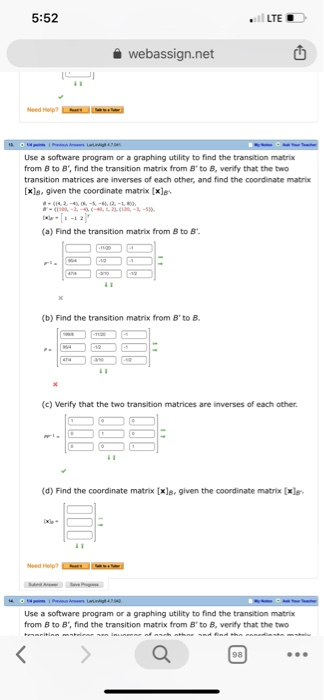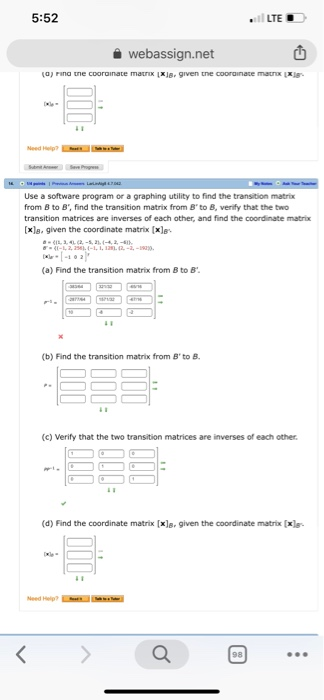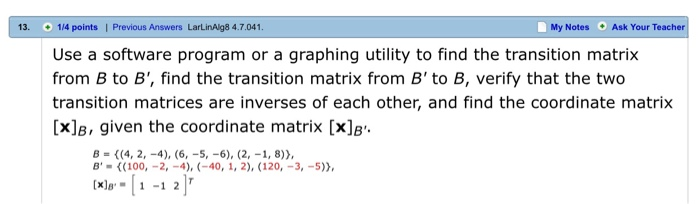5:52 .11 LTE . a webassign.net Use a software program or a graphing utility to find the transition matrix from B to B", find the transition matrix from B' to B, venify that the two transition matrices are inverses of each other, and find the coordinate matrix xls. given the coordinate matrix (xs (a) Find the transition matrix from B to B (b) Find the transition matrix from B' to B (c) Verify that the two transition matrices are inverses of each other (d) Find the coordinate matrix [xls. given the coordinate matrix [xle- Use a software program or a graphing utility to find the transition matrix from B to B", find the transition matrix from B' to B, venity that the two
5:52 LTED webassign.net a rina tne cooroinate maxxl given the coorainate matrx xe Use a software program or a graphing utility to find the transition matrix from B to B', find the transition matrix from B'to B, venify that the two transition matrices are inverses of each other, and find the coordinate matrix [xls. given the coordinate matrix [xs (a) Find the transition matrix from B to B (b) Find the transition matrix from B' to B. (c) Verify that the two transition matrices are inverses of each other (d) Find the coordinate matrix [xls. given the coordinate matrix [xa- a 回
5:52 .11 LTE . a webassign.net Use a software program or a graphing utility to find the transition matrix from B to B", find the transition matrix from B' to B, venify that the two transition matrices are inverses of each other, and find the coordinate matrix xls. given the coordinate matrix (xs (a) Find the transition matrix from B to B (b) Find the transition matrix from B' to B (c) Verify that the two transition matrices are inverses of each other (d) Find the coordinate matrix [xls. given the coordinate matrix [xle- Use a software program or a graphing utility to find the transition matrix from B to B", find the transition matrix from B' to B, venity that the two
13. 1/4 points I Previous Answers LarLinAlg8 4.7.041 My Notes Ask Your Teacher Use a software program or a graphing utility to find the transition matrix from B to B', find the transition matrix from B' to B, verify that the two transition matrices are inverses of each other, and find the coordinate matrix [x]B, given the coordinate matrix [x]B B-((100,-2,-4),-40, 1, 2),(120,-3,-5) (xl 1 -1 2

#### Homework Answers

Answer #1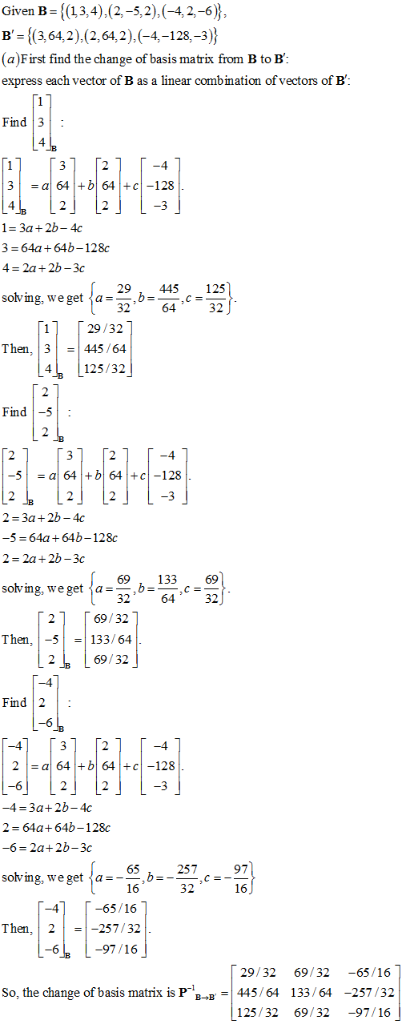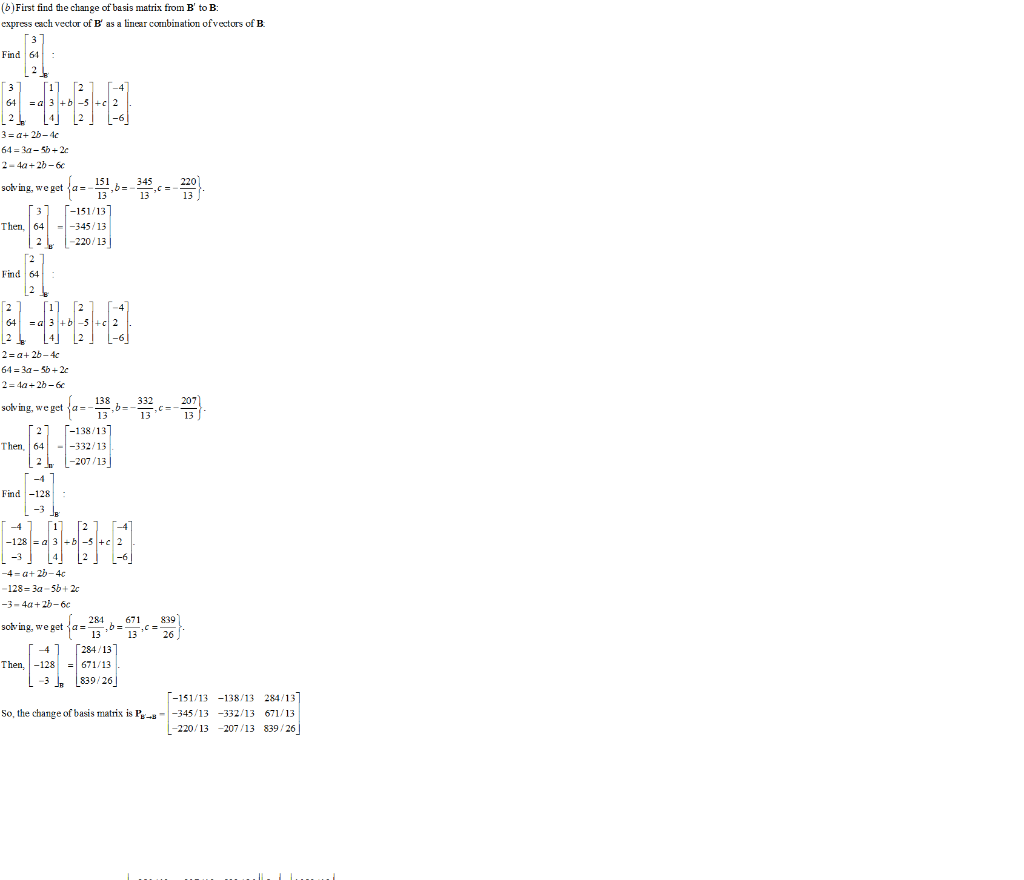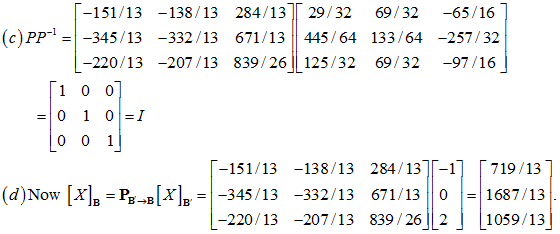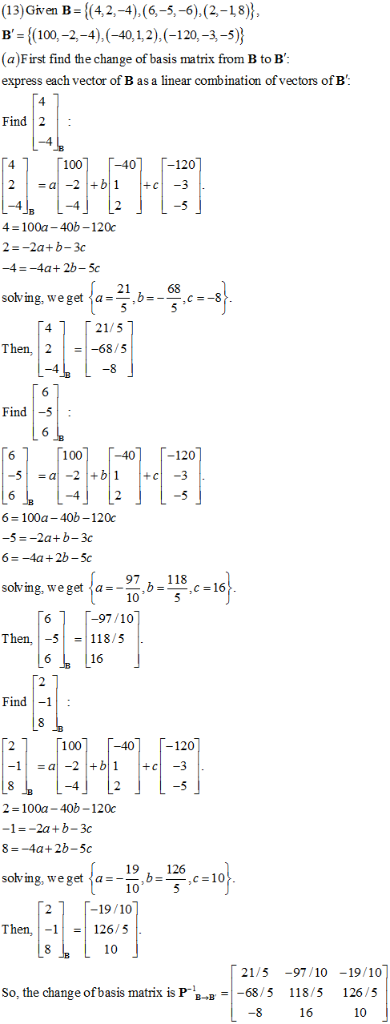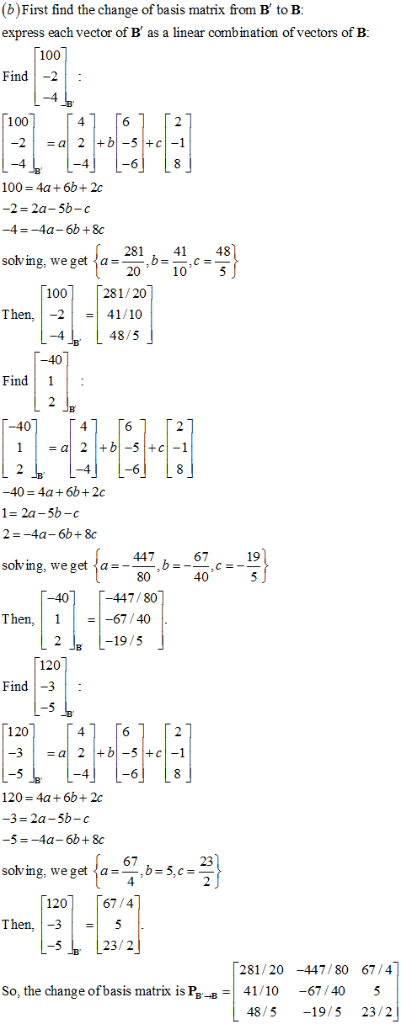Know the answer?
Your Answer:

#### Post as a guest

Your Name:

What's your source?

#### Earn Coin

Coins can be redeemed for fabulous gifts.

Not the answer you're looking for? Ask your own homework help question. Our experts will answer your question WITHIN MINUTES for Free.
Similar Homework Help Questions
• ### 14. 0-4 points LarLinAlg8 4.7.042 My Notes O Ask Your Tea Use a software program or a graphing utility to find the transition matrix from B to B', find the transition matrix from B' to B...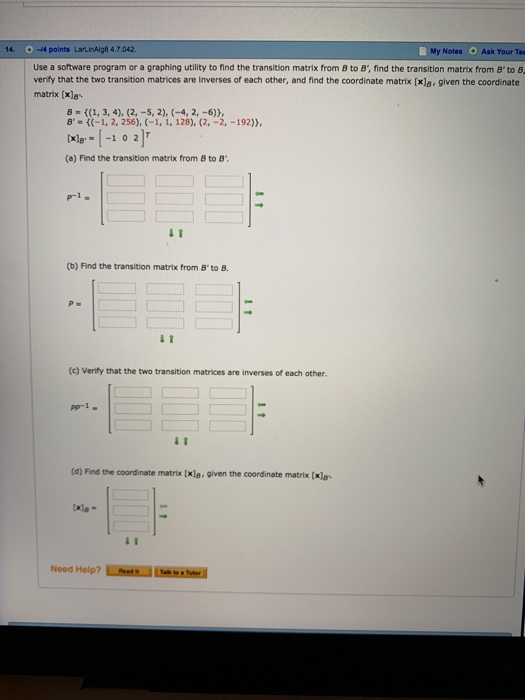14. 0-4 points LarLinAlg8 4.7.042 My Notes O Ask Your Tea Use a software program or a graphing utility to find the transition matrix from B to B', find the transition matrix from B' to B verify that the two transition matrices are Inverses of each other, and find the coordinate matrix [xls, given the coordinate matrix [xle B' = {(-1, 2, 256), (-1, 1. 128), (2,-2,-192)), l102 (a) Find the transition matrix from 8 to B (b) Find the...

• ### Please help. Use a so tware p gram or graphing ut ity to亓nd the transton matnx fom 8 to θ find the trans ton matnx to e verify that the o trans tion matrces are inverses o each other, and the coordi...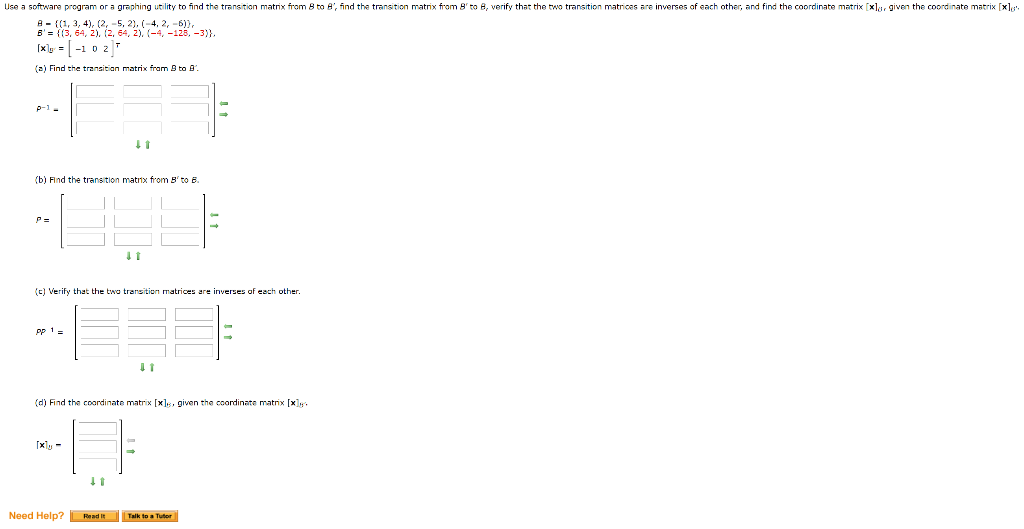Please help. Use a so tware p gram or graphing ut ity to亓nd the transton matnx fom 8 to θ find the trans ton matnx to e verify that the o trans tion matrces are inverses o each other, and the coordinate matrix x c given the coor ate matri om x B 3, 64, 2), (2, 64A, 2), 4, -128, -3) Find the transitian matrix fran S to B (b) Flnd the trans tion matiix from 3 to B....

• ### Use a software program or a graphing utility with matrix capabilities and Cramer's Rule to solve...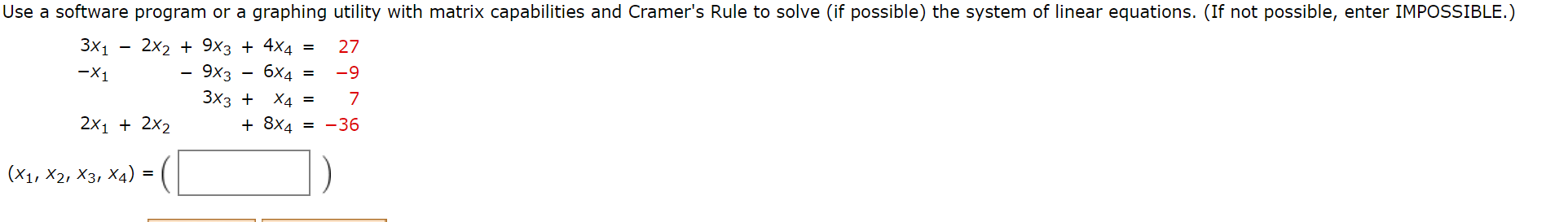Use a software program or a graphing utility with matrix capabilities and Cramer's Rule to solve (if possible) the system of linear equations. (If not possible, enter IMPOSSIBLE.) 3x1 - 2x2 + 9x3 + 4x4 = 27 -X1 - 9x3 – 6X4 = -9 3x3 + X4 = 7 2X1 + 2x2 + 8x4 = -36 (x1, x2, x3, x4) = Use Cramer's Rule to solve the system of linear equations for x and y. kx + (1 - k)y...

• ### Use a graphing utility to find the inverse, if it exists, of the given matrix. Select...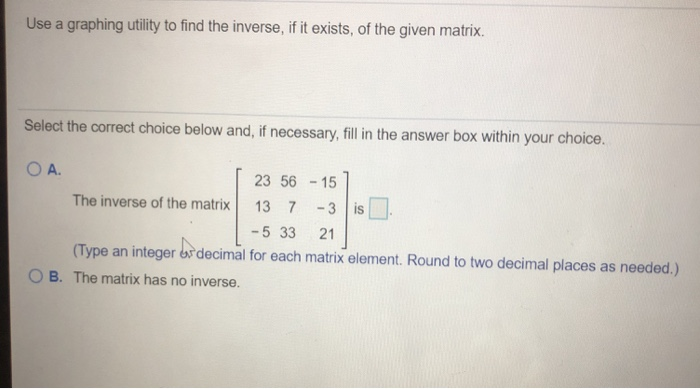Use a graphing utility to find the inverse, if it exists, of the given matrix. Select the correct choice below and, if necessary, fill in the answer box within your choice. OA. 23 56 - 15 The inverse of the matrix 13 7 -3 is . - 5 33 21 (Type an integer os decimal for each matrix element. Round to two decimal places as needed.) OB. The matrix has no inverse.

• ### 6. Find the transition matrix from B to B' and find the coordinate matrix xB', given...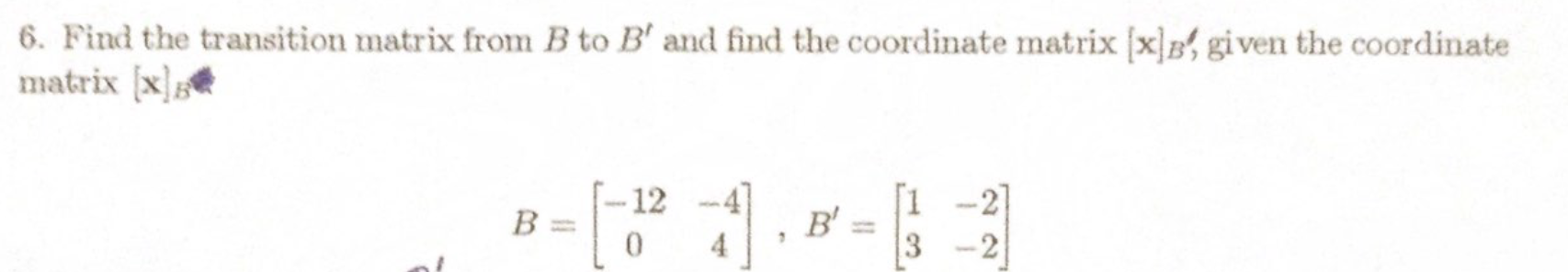6. Find the transition matrix from B to B' and find the coordinate matrix xB', given the coordinate matrix xlge |-12 -4 , B' = 3 - 2 B

• ### (1 point) Consider the ordered bases B = a. Find the transition matrix from C to...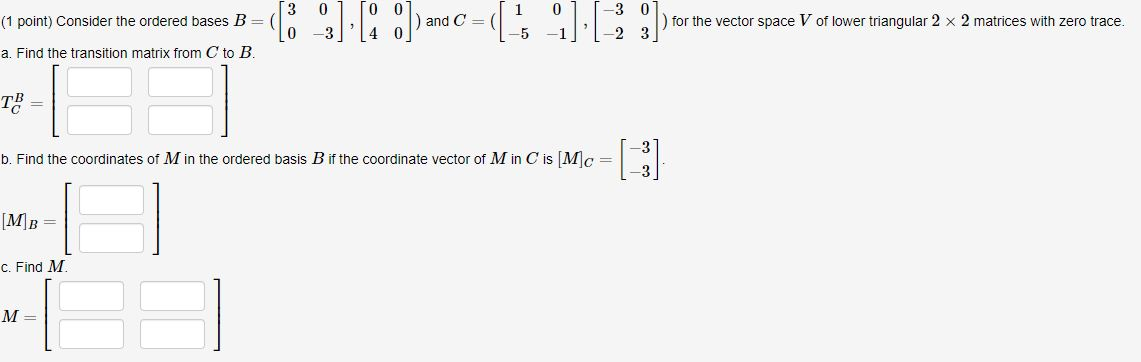(1 point) Consider the ordered bases B = a. Find the transition matrix from C to B. 3 01 To Olmedi 011-3 0. *1 for the vector space V of lower triangular 2 x 2 matrices with zero trace. 3 4 01) and C=-5 -1/'1-23] b. Find the coordinates of M in the ordered basis B if the coordinate vector of M in C is M c [ MB = C. Find M. M =

• ### 10 Consider the two basis B-1,1 of R3 (a) Find matrix that changed the coordinates from the basis...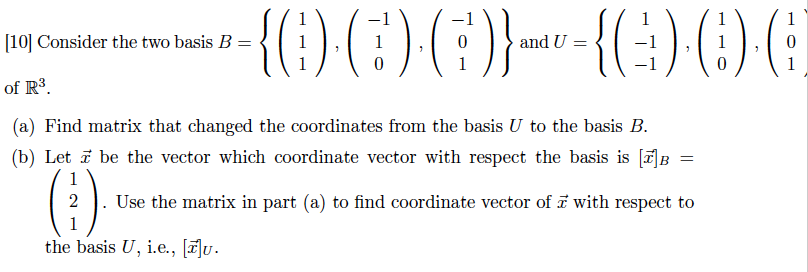Please provide specific explanations with each correct answers. Thanks. 10 Consider the two basis B-1,1 of R3 (a) Find matrix that changed the coordinates from the basis U to the basis B. (b) Let f be the vector which coordinate vector with respect the basis is B- 2. Use the matrix in part (a) to find coordinate vector of with respect to the basis U, i.e., [21. 10 Consider the two basis B-1,1 of R3 (a) Find matrix that changed...

• ### A force of 52 pounds acts on the pipe wrench shown in the figure below 18 in. (a) Find the magnitude of the moment about O by evaluating OA x FIl (O s 0 s 1809) Use a graphing utility to graph th...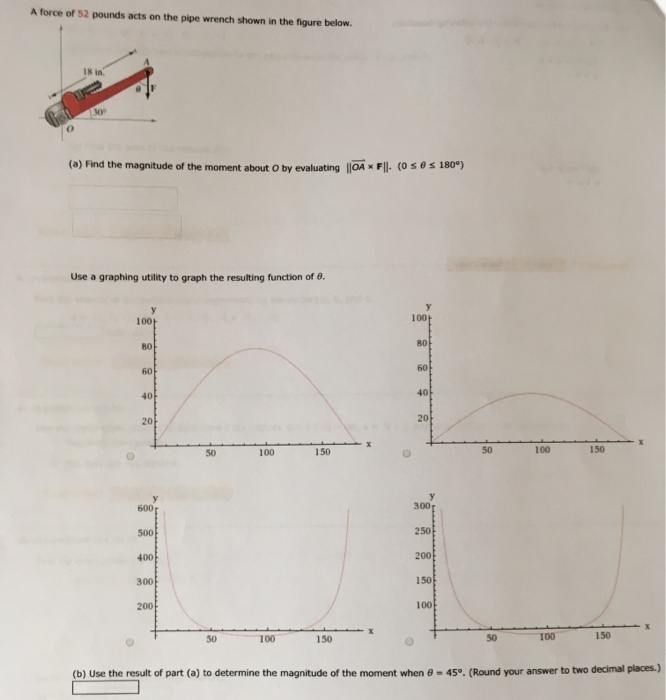A force of 52 pounds acts on the pipe wrench shown in the figure below 18 in. (a) Find the magnitude of the moment about O by evaluating OA x FIl (O s 0 s 1809) Use a graphing utility to graph the resulting function of e 100 100 80 60 40 40 20 20 150 50 100 150 100 300 600 250 500 200 400 150 300 100 200 150 50100 50100 150 Round your answer to two decimal...

• ### Write a MATLAB program that loads two matrices A and B from the files hw41matA.txt andhw41matB.txt...

Write a MATLAB program that loads two matrices A and B from the files hw41matA.txt andhw41matB.txt and multiplies them together to obtain matrix C such that the element C(i,j) ofmatrix C is given by: C(i, j) =  ∑A(i, k) ∗ B(k, j) where n = number of columns in A = number of rows in B. Display the matrix C in defaultMATLAB format. You cannot use the MATLAB matrix multiply or other inbuilt MATLAB functions forarithmetic operations. You must implement it....

• ### Write a c++ program: Many mathematical problems require the addition, subtraction, and multiplication of two matrices. Write an ADT Matrix. You may use the following class definition: const int MAX_RO...

Write a c++ program: Many mathematical problems require the addition, subtraction, and multiplication of two matrices. Write an ADT Matrix. You may use the following class definition: const int MAX_ROWS = 10; const int MAX_COLS = 10; class MatrixType { public: MatrixType(); void MakeEmpty(); void SetSize(int rowsSize, int colSize); void StoreItem(int item, int row, int col); void Add(MatrixType otherOperand, MatrixType& result); void Sub(MatrixType otherOperand, MatrixType& result); void Mult(MatrixType otherOperand, MatrixType& result); void Print(ofstream& outfile); bool AddSubCompatible(MatrixType otherOperand); bool MultCompatible(MatrixType otherOperand);...

Free Homework App

Scan Your Homework
to Get Instant Free Answers
Need Online Homework Help?

Get Answers For Free
Most questions answered within 3 hours.